## Ron90's Content

There have been 15 items by Ron90 (Search limited from 27-November 20)

#### See this member's

Sort by                Order

### #101422ExamPosted by on 27 May 2008 - 09:34 PM in Biology

I found the M/C very good actually. The easiest out off all the past papers. Here are my answers (which i think i have gotten full marks) which have been cross-checked with another person, so there may be mistakes.

1. C 11. B 21. C
2. D 12. D 22. B
3. C 13. A 23. B
4. D 14. A 24. A
5. B 15. D 25. C
6. A 16. D
7. D 17. C
8. A 18. A
9. B 19. A
10. A 20. B

### #101261Exam 2008 Post MortemPosted by on 20 May 2008 - 01:44 PM in Mathematics

Any chance of someone with paper 1 scanning it in?

BTW here is paper 2:

P.1
P.2
P.3

### #101229Good luck!Posted by on 19 May 2008 - 05:52 PM in Mathematics

Yeah Good Luck

Let There Be As Little Log Questions As Possible

I hear that.

### #1012072007 Paper 1 Q9(a)Posted by on 19 May 2008 - 02:26 PM in Mathematics

f(x) = 3x - x^3

(a) Find the exact values where the graph of y = f(x) meets the x- and y-axes.

Ive found where it crosses the y-axis by making the x = 0 and got it as (0,0) which is correct.

However how do u find where it meets the x-axis? (apart from 0,0)

### #101194LogsPosted by on 18 May 2008 - 05:22 PM in Mathematics

I need a couple of things cleared up:

is 10^x the inverse of log(base10)x ?

is e^x the inverse of ln?

Is log(baseE) the same as ln?

### #1011432007 specamin non calc q4 multiple choicePosted by on 16 May 2008 - 04:08 PM in Mathematics

is this the distance between two points question or am i looking at the wrong paper?

yeah. You find BA dont you then use the distance formula?

### #1011412006 paper II Q10(b)Posted by on 16 May 2008 - 04:02 PM in Mathematics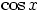andare inverse functions of each other, ie

y =can be changed to x =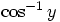and vice versa
you started with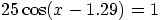divide through by 25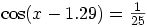take inverse cosines of both sides ie, use the rule above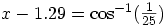then move the 1.29 to the other side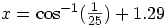hope this helpsBrilliant. Get it now thanks### #1011392007 specamin non calc q4 multiple choicePosted by on 16 May 2008 - 03:57 PM in Mathematics

Well ive got the downloaded version off the SQA website and it gives the answer as C.

Ive done it as well and I got C.

### #1011382006 paper II Q10(b)Posted by on 16 May 2008 - 03:50 PM in Mathematics

Im stuck on this question.

Ive looked at a solution to it and it goes like this:

25cos(x-1.29) = 1

then to

x =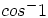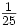+ 1.29

im confused how this is done. Any help?

### #100700More HelpPosted by on 21 January 2008 - 08:32 PM in Mathematics

hmmmm, I got both 50 and 10, is there something in the question that ruled out 10?, and I think you ruled out the one >120 too early, make sure you don't ignore answers that are out of range until the very end, they may end up coming back into rangeOk what you have is right, the graph shows it hits 0 twice before 60 and so that P points to the second one so that makes sense. Thanks!### #100698More HelpPosted by on 21 January 2008 - 08:00 PM in Mathematics

Are you sure you have the correct equation there...I'm getting 2 answersyea i got 2 too, but one is >120 so i crossed that one out.

But the one i had left was 10, and the answer is 50### #100695More HelpPosted by on 21 January 2008 - 07:37 PM in Mathematics

Yeah im again stuck at a question:

(b) Determine the exact value of the
x-coordinate of P, the point where
the graph intersects the x-axis

I have figured out the eq of function is: y = 2Sin(3x)-1 0<x<120

Any ideas? Thanks

### #100685Need Help with QuestionPosted by on 20 January 2008 - 02:01 AM in Mathematics

Thanks a lot, its a bit late for me to be thinking about maths right now, so ill try it in the morning### #100681Need Help with QuestionPosted by on 19 January 2008 - 04:55 PM in Mathematics

I'm also having problems with this Question:

11. (a) Express f(x) = (sq root)3 cos x + sin x in the form kcos (x  a), where k > 0 and
0 < a < pi/2

(b) Hence or otherwise sketch the graph of y = f(x) in the interval 0 ≤ x ≤ 2pi

Thanks again.

### #100680Need Help with QuestionPosted by on 19 January 2008 - 04:16 PM in Mathematics

Seems a really simple question, but I can's seem to be able to work it out:

A function f is defined by the formula f(x) = 3x  x3.

Find the exact values where the graph of y = f(x) meets the x- and y-axes